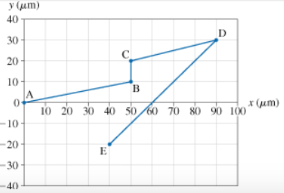# Problem: The bacterium Escherichia coli (or E. coli) is a single- celled organism that lives in the gut of healthy humans and animals. When grown in a uniform medium rich in salts and amino acids, these bacteria swim along zig-zag paths at a constant speed of 20 μm/s. The figure shows the trajectory of an E. coli as it moves from point A to point E. Each segment of the motion can be identified by two letters, such as segment BC.For the segment AB in the bacterium's trajectory, calculate the x component of its velocity.

🤓 Based on our data, we think this question is relevant for Professor Lewis' class at NCSU.

###### FREE Expert Solution

The x-component of velocity in AB:

$\overline{){{\mathbf{v}}}_{\mathbf{A}\mathbf{B}\mathbf{x}}{\mathbf{=}}\frac{\mathbf{A}{\mathbf{B}}_{\mathbf{x}}}{{\mathbf{t}}_{\mathbf{x}}}}$

The length AB is given by:

$\begin{array}{rcl}\mathbf{A}\mathbf{B}& \mathbf{=}& \sqrt{\mathbf{A}{{\mathbf{B}}_{\mathbf{x}}}^{\mathbf{2}}\mathbf{+}\mathbf{A}{{\mathbf{B}}_{\mathbf{y}}}^{\mathbf{2}}}\\ & \mathbf{=}& \sqrt{{\mathbf{50}}^{\mathbf{2}}\mathbf{+}{\mathbf{10}}^{\mathbf{2}}}\end{array}$

AB = 50.99 μm

The angle between AB and the positive x-axis can be found from the graph as:###### Problem Details

The bacterium Escherichia coli (or E. coli) is a single- celled organism that lives in the gut of healthy humans and animals. When grown in a uniform medium rich in salts and amino acids, these bacteria swim along zig-zag paths at a constant speed of 20 μm/s. The figure shows the trajectory of an E. coli as it moves from point A to point E. Each segment of the motion can be identified by two letters, such as segment BC.For the segment AB in the bacterium's trajectory, calculate the x component of its velocity.